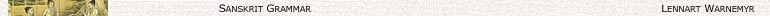Contents

# Reduplication (R)

R is the process of puting a with some changes before itself and it occurs in IIIPr, RAo, Pf, Ds and Int.

# Notation

As usual,

= [1K] A[2K]

and,

 R√ reduplicated root, RK consonant in the reduplicated root, RA vowel of the reduplicated root, 1:1K the first K of 1K, 2:1K the second K of 1K

Two -forms has to be noted:

= 1K A[2K]

 R√√ = RK RA 1K √A[2K]. (1)

= A[2K]

 R√√ = RA RK √A[2K]. (2)

# RK

## √ = 1K √A[2K]

 1K = Ś¬O:2:1K > *RK = 2:1K (3) 1K = Vl: 1:1Ki K > *RK = Pl:1:1Ki (4)

otherwise,

 *RK = 1:1K (5)

 *RK = HKi > RK = ¬HKi (6)

otherwise,

 RK = *RK (7)

## √ = √A[2K]

Except for a few in Ds and two in Int (i, S = īyāya; , S = alar ) this commonly occurs only in Pf.

 RK = ∅ (8)

# III:RA

RK

III:RA is formed as:

 √A = ṝ̆ > III:RA = i (9)

otherwise,

 III:RA = √Ă (10)

# Ao:RA

RK

Ao:RA is formed as:

 √A = ū̆ > Ao:RA = ū (11)

otherwise,

 Ao:RA = ī (12)

 √A is shortened so that RA 1K is prodically long and √A[2K] short (– ∪) (13)

# Pf:RA

RK

## √ = 1K √A[2K]

 √A = ṝ̆ > Pf:RA = a (14) √ = 1K δA > Pf:RA = a (15)

otherwise,

 Pf:RA = √Ă (16)

## √ = √A[2K]

 √ which are prodically long use the periphrastic Pf or: (17) √ = a σK > RS = ā σK (18a) √ = a δK > RS = ān δK (18b) √ = i σK > W:RS = ī σK (19a) S:RS = iye σK (19b) √ = u σK > W:RS = ū σK (20a) S:RS = uvo σK (20b) √ = ṛ σK > RS = ān σK (21)

# Ds:RA

RK

Ds:RA is formed as:

 √A = ū̆ ∨ (Ds-5) > Ds:RA = u (22)

otherwise,

 Ds:RA = i (23)

# Int:RA

RK

 √A = ā̆ ∨ ṝ̆ > Int:RA = ā (24) √A = ī̆ > Int:RA = e (25) √A = ū̆ > Int:RA = o (26)

# Examples

 R-stems √ IIIPr RAo Pf Ds Int dā ‘give’ dadā° adīda° dadā° didā° dādā° hu ‘sacrifice’ juho° ajūha° juhā° juhū° johav° bhū ‘become’ - abībha° babhū° bubhū° bobhav° budh ‘awaken’ - abūbudh° bubudh° bibādh° bābadh jñā ‘know’ - - jajñā° jijñā° jājñā° kṛ ‘do’ - acīkar° cakār° cikīr° (karikṛ°)